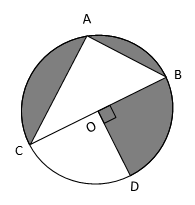# Past CBSE Board Paper

Class 10 Math 2017 Set 3 Q31. Geometry

#### Question In the given figure, O is centre of the circle with AC = 24 cm, AB = 7 cm and ∠BOD = 90°. Find the area of the shaded region.#### Video ExplanationTherefore, CB = $$sqrt {{CA}^{2}+{{AB}^{2}}}$ = $\sqrt {{24}^{2}+{{7}^{2}}}$ = $\sqrt {576+49}$ = $\sqrt {625}$ = 25 BC, the diameter of the circle = 25. So, radius of the circle = $\frac{25}{2}$ Area of shaded region = area of circle – area of right triangle ABC – area of sector COD = π ${\left$ {{$frac {25} {2}}} \right$}^{2}) - $\frac{1}{2}$ × 24 × 7 - $\frac{90}{360}$π ${\left$ {{$frac {25} {2}}} \right$}^{2}) = π ${\left$ {{$frac {25} {2}}} \right$}^{2}) - $\frac{1}{2}$ × 24 × 7 - $\frac{1}{4}$π ${\left$ {{$frac {25} {2}}} \right$}^{2}) = π ${\left$ {{$frac {25} {2}}} \right$}^{2}) - $\frac{1}{4}$π ${\left$ {{$frac {25} {2}}} \right$}^{2}) - $\frac{1}{2}$ × 24 × 7 = $\frac{3}{4}$ π ${\left$ {{$frac {25} {2}}} \right$}^{2}) - $\frac{24 × 7}{2}$ = $\frac{3}{4}$ × $\frac{22}{7}$ × $\frac{625}{4}$ - $\frac{24 × 7}{2}$ = $\frac{20625-4704}{56}$ = $\frac{15921}{56}$ = 284.3$approx)